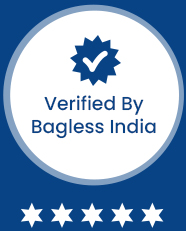### Magical Maths Short Tricks## Magical Maths Short Tricks

• Beginners
• Intermediate
• All
Syllabus Overview
• Math Introduction
• Multiplication
• Multiply any digit by 99
• Multiply any digit by 11
• Square of two Digits
• Square any digit less than 50
• Square Root
• Algebra Part 1
• Algebra part 2
• Cube Root
• Square Root of Decimal Digits
• Division by 9
• Division of Decimal Digits
• Division Method
• Fraction Trick
• Magical Cube Root Trick
• Magical Method for two digit numbers
• Magical tricks for Multiplication
• Multiplication of Number Ending with 1-Type 1
• Multiplication of Number Ending with 1-Type 2
• Pattern Method

Cost of course

6000.00

3599.00

No. of PDF

0No. of videos

21

Live Session

0